# Stability of the swing plane

Dave Tutelman  --  September 19, 2016

I had an email discussion with Todd Dugan about the implications of stability of the swing plane. Todd is a golf instructor and I am an engineer, so the first thing we had to do was to reconcile what "stability" actually means; it has a very specific meaning in mechanics. Ultimately, Todd wanted to make a statement about the putting stroke, but I found it very instructive to start with the full swing. My own takeaway from the discussion is:
• Dynamic forces do a good job of stabilizing the swing plane for a full-swing drive.
• Gravity dominates the forces affecting the putting swing plane; dynamic stability has relatively little to do with it.
Here is how I came to that conclusion.

## Stability and the swing plane

The first step in solving a problem is a precise statement of what the problem is. In this case, both "stability" and "swing plane" need to be defined carefully. The former has a scientific definition that is not exactly the same as the colloquial use, even though it is consistent with it. The latter is controversial among instructors and biomechanists; but we are going to limit the discussion to a part of the swing that everybody agrees is pretty much planar.

### What is stability?

Engineers and physicicsts talk about a concept called "equilibrium". It refers to an object which, when placed in a particular position, will stay in that position. There are three recognized kinds of equilibrium: stable, unstable, and neutral. Wikipedia has an excellent article explaining the difference, and I'm going to borrow a few diagrams from it to present the definition in my own words. Each diagram shows a ball resting on a surface of some shape.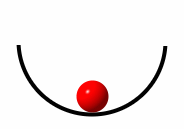Stable equilibrium: If you move the ball left or right, gravity provides a force that wants to return the ball to its original position.Unstable equilibrium: If you move the ball left or right, gravity provides a force that wants to push the ball even further from its original position. The original equilibrium is a delicate balance, not stable at all.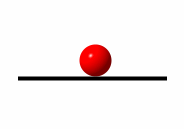Neutral equilibrium: If you move the ball left or right, it will stay where you put it. Gravity does not have a preference for any position on this flat surface.

We will use the term "stability" in this spirit. A swing plane is considered stable if any perturbation of the club from the plane causes a force that wants to return the club on-plane. In fact, that happens -- and to greater or lesser degrees with different kinds of swings.

### What is the swing plane?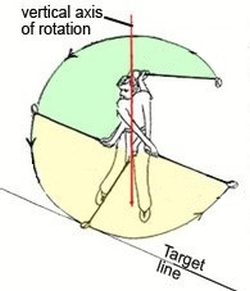I'm going to borrow another diagram to show what I'm using as the definition of the swing plane. There have been many studies that show the downswing not to be on a single plane. And if you've ever watched Peter Kostis draw lines on the SwingVision image on TV, I'm sure you believe that. It's way more complicated than a plane, and probably more complicated than anybody needs to think about. But there is a part of the swing that stays remarkably close to a plane. It is the yellow sector of the circle in the diagram, from shaft-horizontal on the downswing, at least to the ball, and probably to shaft-horizontal on the follow-through. A significant portion of the biomechanics world has dubbed this the "functional swing plane", and there are good physical reasons it is planar. In fact, the reason is stable equilibrium, as we will see.

## Stability of the full-swing plane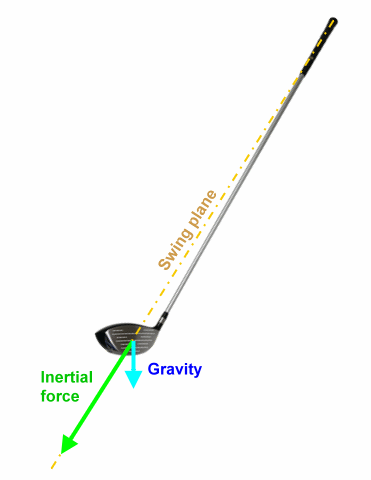The clubhead moves in a plane, the functional swing plane, for the 90° arcs just before and just after impact. Let's look at the dynamics of that part of the swing, to see whether the swing has equilibrium there, and whether that equilibrium is stable, unstable, or neutral.

The diagram shows two forces that the clubhead exerts on the shaft in the vicinity of impact:
• The inertial force in reaction to the pull that the shaft exerts to curve the path of the clubhead. It is an "equal and opposite reaction" (in Newton's terms) to the centripetal force, and is commonly referred to as "centrifugal force". Without getting into a fight about nomenclature or whether the force is "real", it can be usefully quantified. The clubhead is moving on a curved path that is at least approximately planar, and the force must thus act in that plane. Its direction is away from the center of curvature (to be equal and opposite to the pull of the shaft), and its magnitude is:
 Fi  = mv2 R
where:
m is the mass of the clubhead.
v is the velocity of the clubhead.
• Gravity acts in a vertical direction, straight down. Its magnitude is
Fg  =  mg
where:
m is the mass of the clubhead.
g is the acceleration of gravity, a constant. It is 32 feet per second per second in English units and 9.75 meters per second per second in MKS units.

These are the forces that tug on the shaft and on the hands that will either keep the club in-plane or move it out-of-plane. The stability of the swing plane can be measured by comparing the in-plane forces to the out-of-plane forces. If the forces that keep the swing in-plane dominate, then we can say the plane is stable. That is the basis of this investigation!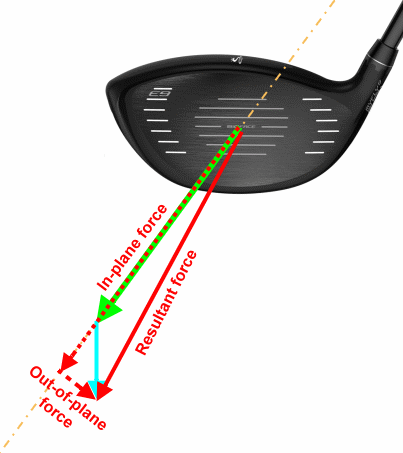To this end, I have made a spreadsheet to compute the in-plane and out-of-plane components of the inertial and gravitational forces. You can download it yourself and play with the numbers if you're not happy with my choice of numbers. The inputs to the spreadsheet are what we have already seen -- m, v, and R -- plus the angle of elevation of the swing plane. In the vicinity of impact, this corresponds to the proper dynamic lie of the golf club.

The diagram illustrates two ways of thinking about this stability figure of merit. They are mathematically identical, just different ways you can view it.
1. The ratio of the in-plane force to out-of-plane force. (Those are the dotted red force vectors.) The larger this number, the more stable the swing plane. A large number means that the forces that want to restore the clubhead on-plane dominate the forces that want to move it off-plane.
2. The angle between the resultant force and the swing plane. (The resultant is the solid red force vector.) That shows just how far out-of-plane is the total force on the clubhead. If the angle is small, then the forces are close to the plane and the clubhead will stay more or less on-plane.
Why do I say they are mathematically identical? The ratio of in-plane to out-of-plane force (figure of merit #1) is the cotangent of the angle between the resultant and the swing plane (figure of merit #2). So from either one you can easily get the other.

Notice that the diagram includes both the inertial force (green) and the gravity force (blue). Most of that is in-plane, but at least part of the gravity force is out-of-plane.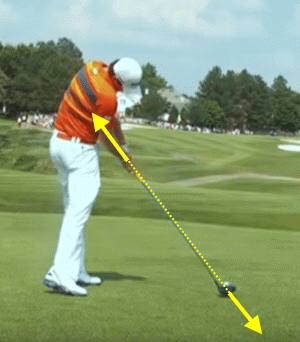I want to belabor the point, so that it is completely clear why stability is governed by the relationship between in-plane and out-of-plane forces. Look at the picture of Rory McIlroy, one of the best drivers of the golf ball, near impact. I have added the forces at the two ends of the shaft: Rory pulling in on the shaft with his hands, and the clubhead pulling out on the shaft.

If the two forces are not in the same line (that line being the shaft axis), then they are parallel. What does that mean? They will form a moment -- a torque -- equal to the magnitude of the forces times the distance between them. If they are not along the same axis, the resulting torque will tend to turn the club so that the forces do line up. If you look again at the defintion of stable equilibrium, that is exactly what it is about. If the forces are parallel to the plane but not in the plane, they will turn the club to move it back into the plane.

Now that's the in-plane force; it stabilizes the swing plane. But suppose the force has an out-of-plane component. That will tend to accelerate the clubhead away from the swing plane. It does not have any tendency to provide stable equilibrium to the swing plane, unlike the in-plane force. If the out-of-plane force is tiny, if it is dominated by the in-plane force, then the overall effect is a stable swing plane. But, to the extent that the out-of-plane force is at least a significant fraction of the in-plane force, either the club will go out of plane or the hands will have to provide some [beta] torque to keep the club on plane to make good impact with the ball.

Finally, let's get to some numbers. I used the formulas (which are implemented in the spreadsheet) to compute the inertial force Fi, the gravity force Fg, and how they align with the swing plane. I used a driver swing, with the 200-gram clubhead moving at 100mph at impact. I placed the center of rotation of the clubhead's path a few inches above the hands, and the functional swing plane at 50° from horizontal. What I found was:
 Inertial force = 71 pounds Gravity force = 0.4 pounds
That certainly suggests that inertia dominates the forces the clubhead exerts on the shaft. So we should expect this to be a very stable swing plane. And it is. When we resolve in-plane and out-of-plane forces, we find:
 In-plane force = 71 pounds Out-of-plane force = 0.3 pounds In-to-out ratio = 252 Resultant angle off-plane = 0.23°

So the in-plane forces are more than 250 times as large as the out-of-plane forces, and the resultant force is aligned within less than a quarter degree of the swing plane. Conclusion: a very stable swing plane!

One more point worth making: These numbers are a snapshot of the swing plane at impact. That is where the velocity is highest and the clubhead path is likely the most curved. (Not necessarily the most curved part, but you have to do something special to flatten out the curve near impact.) So this is almost certainly the most stable instant of the swing. If we averaged the stability over the entire functional plane, it would be somewhat less stable -- though still dominated by the inertial in-plane forces.

## Stability of the putting swing plane

Now let's look at the putting swing. Does the same thing hold true? Is the putting plane kept stable by inertial forces?Todd's motivation for the question is based on whether the "straight back, straight through" putting stroke is physically sound. If inertia effectively stabilizes the putting swing plane, then the most stable swing would be an "around" swing, not a straight line. Todd's geometric reasoning is perfect; see the diagram for this discussion.
• If the stroke is stabilized by inertia, then the hands have to move in the same plane as the putter head.
• A putter lie is typically in the 70°-75° range -- tilted, not vertical. So that plane must be tilted.
• The putter head (and hands) swing on arcs in the plane. Since the plane is tilted, the horizontal movement of the putter head (the projection of putter head movement onto the ground) cannot be a straight line. It is in-to-square-to-in.
So the only question that remains is the inertial stability of the putting plane. If inertial forces do a good job of stabilizing the plane, that is a fairly strong argument against a putting stroke that is straight back, straight through. It can still be a rocking motion in a single plane. But the plane is tilted and not vertical, so the horizontal component of the path is not straight.

Let's dive in and look at the numbers. A typical putter has a 320-gram head, though there are plenty with heavier heads around. (Actually, while clubhead mass will affect the forces, it will not change the ratios nor angles of those forces; so the choice of head weight is not going to make a difference.) The typical putter lie angle is 72°, so we will use that for the angle of the functional swing plane. And let's assume the putter stroke is a pendulum from the shoulders, so the radius of curvature is about 60 inches.

The most interesting input is the clubhead speed. Also the most critical, since inertial force depends on velocity squared. I happen to have previously computed the ball speed for a fairly typical putt, a 10-foot putt on a green that stimps at 10. That came out to 5mph. So the clubhead speed is 5mph divided by the smash factor. When you plug putter head numbers (mass=320g, COR=.77) into the formula for smash factor, you get 1.55 for the factor and 3.23mph for the clubhead speed.

Here is what the spreadsheet tells us:
 Inertial force = 0.1 pounds Gravity force = 0.7 pounds
Hmmm! This time, the inertial force is considerably smaller that the force due to gravity. That is not a strong argument for stable equilibrium. Let's resolve in-plane and out-of-plane forces, as a check on that:
 In-plane force = 0.77 pounds Out-of-plane force = 0.22 pounds In-to-out ratio = 3.5 Resultant angle off-plane = 16°

The in-plane forces are bigger than out-of-plane. But the ratio is less than 4:1 -- hardly a domination. And the resultant force is 16° out of the swing plane; that suggests the plane is not going to be stable.Wait! How does this even happen? The inertial force was much lower than gravitational force. How did we ever get more in-plane force than out-of-plane? The diagram of forces tells the story.

Yes, the inertial force (green) is considerably smaller than the gravitational force (blue). But the tilt of the swing plane is only 18° from vertical. (That's 90° vertical minus 72° lie angle.) So most of the gravitational force is in the same direction as the shaft axis -- nominally in-plane. That is how we got a larger in-plane force. But it does not suggest a stable swing plane. In fact, the stable plane is with the club hanging nearly vertical.

You can see this when you look at the angles. The resultant force is 16° off-axis. That means there's a lot of force tending to kick the club out of plane. Most telling is the fact that the plane itself it tilted 18°; if the resultant force is 16° from the plane, it is only 2° from straight up and down.

Bottom line: the putter wants to hang vertically. Inertial forces are only helping with 2° out of an 18° deficit. That is not a swing plane with stable equilibrium -- not even close.

## Comparisons and conclusions

Here is the data -- the raw numbers -- from the two cases I've run on the spreadsheet.

 Description Mass (grams) Speed (mph) Radius (inches) Lie Angle (deg) Dynamic Force (lb) Gravity Force (lb) In-plane Force (lb) Out of plane Force (lb) Force ratio Resultant angle from plane (deg) Driver 100mph 200 100 50 50° 70.7 0.439 71.071 0.282 252.1 0.227° 10ft putt, stimp 10 320 3.23 60 72° 0.098 0.702 0.766 0.217 3.531 15.81°

It is clear from these numbers that the driver swing plane enjoys stable equilibrium, while the putting stroke has a lot less stability. A good look says that the putting stroke has almost no stability; there is seven times the tendency to hang straight down as to stay on-plane.

What does this say about the viability of a straight back straight through putting stroke, compared to a tilted-plane putting stroke? Let's return to the picture of the swinging putter head. But now we'll look at two possibilities: a curved path and straight back straight through.Curved path We've already see this one. There is a swing plane that contains the shaft, and obviously both the hands and the putter head. The projection of the putter head's path on the ground is curved -- in to square to in.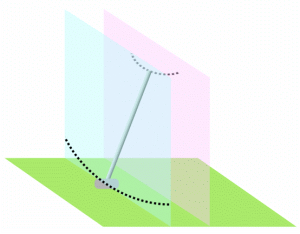Straight back, straight through This stroke has the putter head moving in one plane (the blue vertical plane) and the hands in another (the pink vertical plane). This can be accomplished with a shoulder rock; this time the rocking is in a vertical plane not a tilted one. The result is a putter head path that is straight back and straight through.

This says that there is no argument based on stable equilibrium to favor one style over another. One may in fact be better; I don't know. But stability of the swing plane cannot give us the answer.

Slightly generalizing this conclusion... During our discussion, Todd tried out the principle, "The conditions resulting from an in-plane swing establishes it as most proper; the golfer should always reproduce this condition, even in low-power swings where he may have to provide the restoring force himself."

The words "most proper" and "should" may be a hint that this is a value judgement, not an absolute. The principle may be true. Or it may be false. Or it may be true for some golfers and not others. Or it may even have some truth and some falsehood for every golfer.

There may even be a way to determine whether it is true, false, or something else.
Anatomy? Maybe.
Motor learning? Maybe.
Biomechanical experiments? Maybe.
But the physics of stable equilibrium simply doesn't help us with that question.